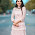### Ticker

6/recent/ticker-posts

# Curves in Alignment of Highways – Different Types of Curves

What is a Curve in Roads?

A curve is nothing but an arc which allow to connects two straight lines which are separated by some certain angle called as deflection angle. This situation occurs where the alignment of a road way or rail way changes its direction due to unavoidable objects or land conditions and many more issue. The object may be a hilly area, home, agricultural land, environmental factors, lake or a temple etc. The curve is provided for the ease of movement of vehicle at point of curve.

Types of Curves in Alignment of Highways

There are two types of curves

1.Horizontal curves

2.Vertical curves

3.Horizontal Curves

The curve provided in the horizontal plane of ground or earth is called as horizontal curve. It connects two straight lines which are in same level but having different or same directions.Curves in Alignment of Highways

Horizontal curves are of different types as follows:
1.Simple circular curve
2.Compound curve
3.Reverse curve
4.Transition curve
5.Spiral
6.Lemniscate

Simple Circular Curve
Simple circular curve is normal horizontal curve which connect two straight lines with constant radius.

Compound Curve
Compound curve is a combination of two or more simple circular curves with different radii. In this case both or all the curves lie on the same side of the common tangent.

Reverse Curve
Reverse curve is generated when two simple circular curves bending in opposite directions are meet at a signle point and that points is called as point of reverse curvature. The center of both the curves lie on the opposite sides of the common tangent such that the radii of both the curves may be same or different.

Transition Curve
A curve of variying radius is termed as transition curve. It is generally provided on the sides of circular curve or between the tangent and circular curve and between two curves of compound curve or reverse curve etc. Its radius varies from infinity to the radius of provided for the circular curve.
Transition curve helps gradual introduction of centrifugal force by gradual super elevation which provides comfort for the passengers in the vehicle without sudden jerking.

Spiral Curve
Spiral is a type of transition curve which is recommended by Indian Road Congress as ideal transition curve because of its smooth introduction of centrifugal acceleration. It is also called as clothoid.

Lemniscate
Lemniscate is a type of transition curve which is used when the deflection angle is very large. In lemniscate the radius of curve is more if the length of chord is less.

Vertical Curves
The curves provided in vertical plane of earth is called as vertical curve. This type of curves are provided when the ground is non-uniform or contains different levels at different points. In general parabolic curve is preferred as vertical curve in the vertical alignment of roadway for the ease of movement of vehicles. But based on the convexity of curve vertical curves are divided into two types

1.Valley curve
2.Summit curve

Valley Curve
Valley curve connects falling gradient with rising gradient so, in this case convexity of curve is generally downwards. It is also called as sag curve.

Summit Curve
Summit curve connects rising gradient with falling gradient hence, the curve has its convexity upwards. It is also called as crest curve.

1.